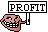This site uses cookies to improve your browsing experience. By continuing to browse the website, you accept such cookies. For more details and to change your settings, see our Cookie Policy and Privacy Policy.

# Math question

•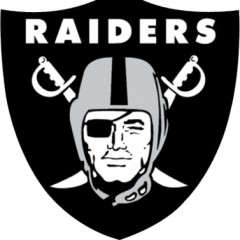Coach
Coach
Joined: 09.07.2010
Odds for team A to win is 1.75
Odds for thing B to happen, if team A wins is 2.1

How often does thing B need to happen, for the bets to be breakeven?
(e.g. how often does thing B need to happen to that the second bet is more profitable than the first?)
• 10 replies
•Bronze
Joined: 01.05.2012
The formula I've been told for evaluating the value of sports bets is:

(decimal bookie's odds) * (chances of winning in percent) / 100 = Profitability (with 1 being break-even, anything above winning, and anything below losing)

Following that, we could replace chances of winning in percent with x and profitability with 1, leading to:

(decimal bookie's odds) * x / 100 = 1

Which leads to:

x = (1 * 100) / (decimal bookie's odds)

In the example, this would lead to a required chance of winning of around 57.14% for Team A to break even, and around 47.62% for Thing B, if I'm not mistaken.

To answer the question, we could replace the chance of Team A with x and the chance of Thing B with y, and put the formula as:

1.75 * x / 100 = 2.1 * y / 100

My algebra is a bit rusty, but I believe it leads to:

x = 1.2 y, suggesting that Thing B needs to happen 1.2 times as often as Team A winning, in order to break even relative to the Team A bet. Can anyone check this?

Unless Thing B in your example is something that needs to happen in addition to Team A winning, in which case we need to adjust the formula.
•Coach
Coach
Joined: 09.07.2010
Yes, B cant happen if team A doesn't win. Could it be just B/A?
•Bronze
Joined: 12.10.2011
Disclaimer: not a math expert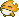Not sure if I fully understand the question but I'll give it a shot. I assume we place both bets at the same time? If so we need to take multiple things into account to find the breakeven point, with the chance of team A winning playing a huge role in how often B needs to happen for both bets to break even. As Harrier said:

The bet for team A is break-even if A wins roughly 57.1% of the time.
The bet for thing B is break-even if B happens roughly 47.6% of the time.

However the bet on B happening is dependent on the bet on A winning. After all, if A loses, it doesn't matter how often B happens; we always lose both bets.

Profits of possible outcomes are as follows:
Profit(A loses) = -\$20
Profit(A wins, B doesn't happen) = \$7.50 - \$10 = -\$2.50
Profit(A wins, B happens) = \$17.50 + \$21 = \$38.50
That allows us to create the following equation:
EV = [1 - P(A wins)] * -\$20 + [P(A wins) * [1 - P(B happens)]] * -\$2.50 + [P(A wins) * P(B happens)] * \$38.50
EV = -20 + 20x -2.50x + 2.50xy + 38.50xy (x is P(A wins), y is P(B happens)
EV = 40.5xy + 17.5x - 20
And then how often B needs to happen to be breakeven depends on the actual probability of team A winning. So say team A wins 100% of the time then the bets are breakeven if:
40.5y + 17.5 - 20 = 0
40.5y = 2.50
y = .0617 = 6.2%
So if A always wins, B needs to happens 6.2% of the time for the bets to be breakeven. You could apply this logic to any 'winrate' for team A.

Interestingly, A needs to win 35% of the time or more to even have a chance of making a profit, although B needs to happen almost 98% of the time in that case for the bets to be breakeven. If A only wins 34% of the time or less, we make a loss even if B happens 100% of the time.

So we can draw the following conclusions:
- If A wins 34% of the time or less, we always make a loss.
- If A wins 35% of the time or more, but B happens less than 6.2% of the time, we always make a loss
- If A wins 35% of the time or more, and B happens more than 98% of the time, we always make a profit

For the chance of B happening between 6.2% and 98%, how often B actually needs to happen for the bets to be breakeven depends very heavily on the actual chance of team A winning and can be found using the above method.

Long story short: given that we have no information besides payouts (odds) there isn't a single break-even point; there are instead many different ones

I hope this makes sense (and I hope I'm not wrong lol)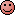•Bronze
Joined: 12.10.2011
x = 1.2 y, suggesting that Thing B needs to happen 1.2 times as often as Team A winning, in order to break even relative to the Team A bet. Can anyone check this?
Assuming my logic is correct, this statement is not true. We see that if team A wins 100% of the time, B needs to happen 6.2% of the time (.062 times as often) and if A wins 35% of the time, B needs to happen 98% of the time (2.8 times as often) to break even. If A wins 80% of the time for example, B needs to happen ~18.5% of the time (.23 times as often).
•Coach
Coach
Joined: 09.07.2010
I need a number that is the breaking point, if I should bet for team A to win, OR thing B to happen. And B can't happen unless team A wins.
•Bronze
Joined: 01.05.2012
Originally posted by TJtheTJ
x = 1.2 y, suggesting that Thing B needs to happen 1.2 times as often as Team A winning, in order to break even relative to the Team A bet. Can anyone check this?
Assuming my logic is correct, this statement is not true.
I assumed that Team A winning and Thing B happening were two independent events (i.e. Thing B does not require Team A to win first).

As far as I know, the likelihood of two cumulative events (e.g. tails on a coin flip plus six on a dice roll) is calculated as chance of A * chance of B. So in the coin flip plus dice roll example, it would be 1/2 * 1/6 = 1/12, or around 8.33%.

Using this and the formula from my last post, we could arrive at something like:

1.75 * x = 2.1 * (x * y)

Wouldn't that result in x cancelling itself out, though?

idk, I'm at the end of my line here.
•Coach
Coach
Joined: 09.07.2010
No no no. We assume that I can make only one bet. Either put my money on team A to win, OR I put my money on thing B to happen (which requires team A to win). How often thing B needs to happen (if A wins) so that it's better option than to bet for team A to win?
•Bronze
Joined: 12.10.2011
Aaah ok, so I completely misunderstood your questionI thought you were betting on both, but you want to know which of the two to bet on. In that case yes, Harrier is going somewhat in the right direction but it's not quite rightMathematical proof below:

EV(A) = P(A wins) * 1.75*bet - [1 - P(A wins)] * bet
EV(B) = P(A wins) * [P(B happens) * 2.10*bet - [1 - P(B happens)] * bet] - [1 - P(A wins)] * bet

Substituting as follows and assuming a bet of 1:
x = P(A wins)
y = P(B happens)

Then:

EV(A) = 1.75x - 1 + x = 2.75x - 1
EV(B) = x * [y * 2.10 - (1 - y)] - 1 + x] = 3.10xy - 1

We want to know when EV(B) > EV(A), so:

3.10xy - 1 > 2.75x - 1
3.10xy > 2.75x
3.10y > 2.75
y > 0.89

To verify:
Assume team A wins 80% of the time:
EV(A) = 2.75 * .8 - 1 = 1.2
EV(B) = 3.10 * .8y - 1 = 2.48y - 1
2.48y - 1 > 1.2
2.48y > 2.2
y > .89

Assume team A wins 50% of the time:
EV(A) = 2.75 * .5 - 1 = 0.375
EV(B) = 3.10 * .5y - 1 = 1.55y - 1
1.55y - 1 > 0.375
1.55y > 1.375
y > .89

Assume team A wins 20% of the time:
EV(A) = 2.75 * .2 - 1 = -0.45
EV(B) = 3.10 * .2y - 1 = .62y - 1
.62y - 1 > -0.45
.62y > 0.55
y > .89
So long story short, B needs to happen more than 89% of the time in order for it to be the better bet, regardless of how often team A wins.

That's the breaking point you're looking for•Coach
Coach
Joined: 09.07.2010
Ok. So instead of betting for Patriots to win, everyone should bet for Brady getting MVP.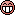•Bronze
Joined: 12.10.2011
Go for it